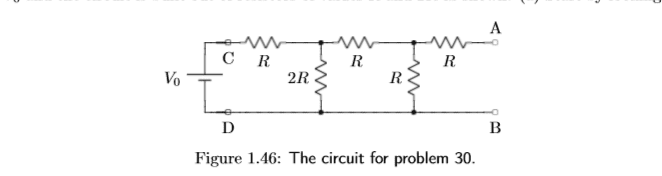# Thevenin and Norton Equivalents

• pestydestroyer

#### pestydestroyer

Hello physics community!

This is my first post to PF and I hope to contribute much more as time goes on. I am having serious trouble understanding Thevenin and Norton equivalent circuits, more specifically on how to find a circuit's equivalent. It only seems appropriate that to find a circuits Thevenin or Norton resistance, you combine or reduce the resistors in the circuit down to one. However, this does not seem to work for many problems, and I realized I don't understand how to find an equivalent circuit at all. My professor said to find the Thevenin voltage, I was to measure the voltage across some output terminals in the circuit, but that gives me no indication on how to go about actually solving. Attached is a picture of some circuit I found and I am trying to find it's Thevenin equivalent. Any help or just an explanation of Thevenin/Norton equivalents would be greatly appreciated. Thanks!Last edited:
You understand the idea correctly, to reduce the resistive network down to one resistor but you did not say what you are supposed to do with any voltage and current sources in the circuit while you are doing it. Also, your belief that "it doesn't work for some problems" is mistaken. More likely it's just that you have not yet figured out how to do it for those problems.

You are supposed do show some attempt at your own work. Find out what it is that you are supposed to do with the sources, if you don't already know, then do that and reduce the resistances down to one, showing how you did it.

When I am told to find the Thevenin voltage, I am told to find the voltage across a and b. I can use Kirchhoff's loop rule to solve for currents throughout the circuit but how do I find the voltage across a and b? Do I say there is some load resistance attached? That doesn't seem to make sense if the Thevenin voltage is dependent on some arbitrary load.

You are not answering my question. What are you supposed to do with voltage and current sources when you are finding the Thevinin equivalent resistance? The answer to that question is fundamental and until you understand it, continuing is pointless.

I believe my problem is that I know how to solve for the Thevenin resistance(open/short all voltage/current sources) but then what do I do with the Thevenin voltage? If I have reduced down a circuit to one resistor, how is the Thevenin voltage not the original voltage?

I know that I am supposed to short/open sources when finding the equivalent resistance, but I am not fully sure why. I think it is this misunderstanding that causes me confusion after finding the equivalent resistance, because it is after that where I have no idea how to move forward.

Okay, so I have the Thevenin resistance and if Vth=Rth*In where In is the norton current across ab if we were to just connect a to b with the same wire being used(in this case with zero resistance), then if I solve the entire circuit for the currents and I find the current across that a-b wire, will that be In?

I know that I am supposed to short/open sources when finding the equivalent resistance, but I am not fully sure why. I think it is this misunderstanding that causes me confusion after finding the equivalent resistance, because it is after that where I have no idea how to move forward.
OK, now we are getting somewhere.

The point of finding the Thevinin equivalent circuit is to simplify a portion of a circuit, such as the entire circuit you have shown but which could be part of a more complex circuit. This simplification has to provide an equivalent that looks to the rest of the world exactly like what it is replacing.

That means it will need to things, a source and a resistance.

The FIRST thing to do is find the resistance seen from the points specified. To do that you replace sources with just their internal resistance and remove the voltage or current. Ideal voltage sources have zero internal resistance and ideal current sources have infinite internal resistance, so that's what you replace them with.

Then, having found the equivalent resistance, you work a different problem to find the equivalent voltage. That problem just amounts to taking the circuit you are finding the equivalent of and figuring out what voltage it presents to the rest of the world at the specified points. This is done without considering the equivalent resistance you just found, just analyze the circuit as is.

EDIT: you posted 2 more posts while I was answering the first one. Best you should use EDIT rather than a new post because when you use edit, it shows up to someone answering you that the post they are answering has been edited. This avoids some confusion.

•pestydestroyer
I'm gone for the night. If you still have questions, perhaps someone else will take up this thread.

I'm gone for the night. If you still have questions, perhaps someone else will take up this thread.
Thank you so much. You helped me understand more in the last 30 minutes than I have so far this semester. And thank you for the post etiquette, I wasn't aware of it.